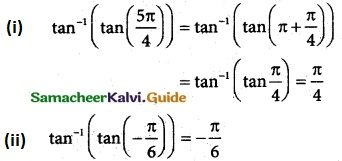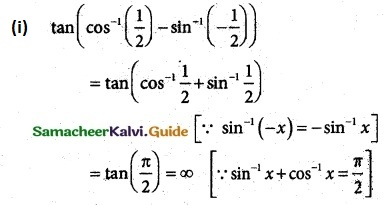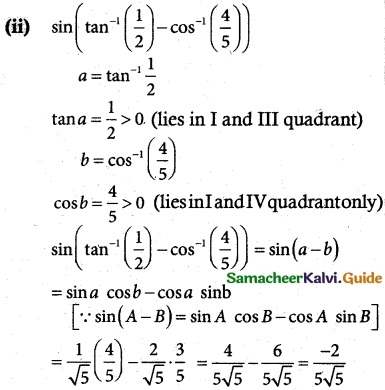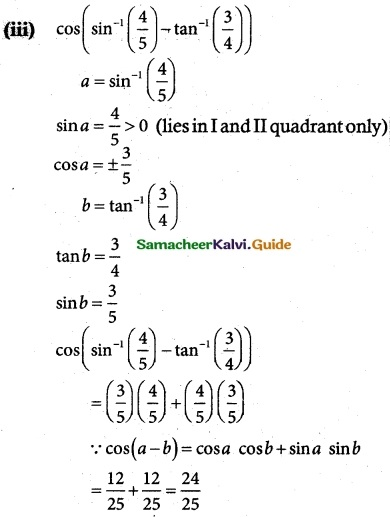Tamilnadu State Board New Syllabus Samacheer Kalvi 12th Maths Guide Pdf Chapter 4 Inverse Trigonometric Functions Ex 4.3 Textbook Questions and Answers, Notes.

## Tamilnadu Samacheer Kalvi 12th Maths Solutions Chapter 4 Inverse Trigonometric Functions Ex 4.3

Question 1.
Find the domain of the following functions
(i) tan-1 ($$\sqrt {9-x^2}$$)
(ii) $$\frac {1}{2}$$ tan-1 (1 – x²) – $$\frac {π}{4}$$
Solution:
(i) f(x) = $$\tan ^{-1}(\sqrt{9-x^{2}})$$
We know the domain of tan-1 x is (-∞, ∞) and range is $$\left(-\frac{\pi}{2}, \frac{\pi}{2}\right)$$
So, the domain of f(x) = $$\tan ^{-1}(\sqrt{9-x^{2}})$$ is the set of values of x satisfying the inequality
$$-\infty \leq \sqrt{9-x^{2}} \leq \infty$$
⇒ 9 – x2 ≥ 0
⇒ x2 ≤ 9
⇒ |x| ≤ 3

(ii) Range of tan-1 x is R
-∞ < 1 – x² < ∞
-∞ < -x² < ∞
-∞ < x < ∞
x ∈ R
Domain = RQuestion 2.
Find the value of
(i) tan-1(tan$$\frac {5π}{4}$$)
(ii) tan-1(tan(-$$\frac {π}{6}$$))
Solution:Question 3.
Find the value of
(i) tan(tan-1($$\frac {7π}{4}$$))
(ii) tan(tan-1(1947))
(iii) tan(tan-1(-0.2021))
solution:
We know that tan(tan-1 x) = x
(i) $$\tan \left(\tan ^{-1} \frac{7 \pi}{4}\right)=\frac{7 \pi}{4}$$
(ii) tan(tan-1(1947))= 1947
(iii) tan(tan-1 (-0.2021)) = -0.2021Question 4.
Find the value of
(i) tan(cos-1($$\frac {1}{2}$$) – sin-1(-$$\frac {1}{2}$$))
(ii) sin(tan-1($$\frac {1}{2}$$) – cos-1($$\frac {4}{5}$$))
(iii) cos(sin-1($$\frac {4}{5}$$) – tan-1($$\frac {3}{4}$$))
solution: